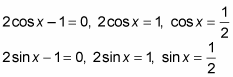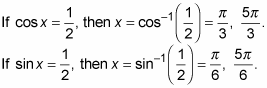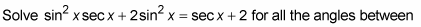##### Trigonometry Workbook For DummiesThe process of factoring by grouping works in very special cases, when the original trigonometry expression is the result of multiplying two binomials together that have some unrelated terms in them. You usually can apply this type of factoring when you’re facing an even number of terms and can find common factors in different groups of them.

The types of equations that you can solve by using grouping look like 4sin x cos x – 2sin x – 2cos x + 1 = 0 or sin2 x sec x + 2sin2 x = sec x + 2. In the first equation, the first two terms have an obvious common factor, 2sin2 x. The second two have no common factor other than 1, but to make grouping work, you will factor out –1.

Solve 4sin x cos x – 2sin x – 2cos x + 1 = 0 for all the possible answers between 0 and 2π.

1. Factor 2sin x out of the first two terms and –1 out of the second two.

2sin x (2cos x – 1) – 1(2cos x – 1) = 0

Now you have two terms, each with a factor of 2cos x – 1.

2. Factor that common factor out of the two terms.

(2cos x – 1)(2sin x – 1) = 0

3. Set the two factors equal to 0.4. Solve for the values of x that satisfy the equation.This next example of grouping requires that you begin by moving the two terms on the right to the left. Another twist is that one of the resulting factors turns out to be a quadratic. How can math be much more fun than this?0 and 360 degrees.

1. Move the terms on the right to the left by subtracting them from both sides.

sin2 x sec x + 2sin2 x – sec x – 2 = 0

2. Factor sin2 x out of the first two terms and –1 out of the second two.

sin2 x (sec x + 2) – 1(sec x + 2) = 0

3. Now factor sec x + 2 out of the two terms.

(sec x + 2)(sin2 x – 1) = 0

4. Set the two factors equal to 0.

sec x + 2 = 0, sec x = –2

sin2 x – 1 = 0, sin2 x = 1, sin x = 1 when you take the square root of both sides.

5. Solve for the values of x that satisfy the equations.

If sec x = –2, then x = sec–1(–2) = 120º, 240º.

If sin x = 1, then x = sin–1(1) = 90º.

If sin x = –1, then x = sin–1(–1) = 270º.